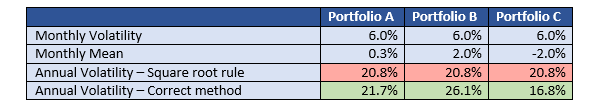A common task in the performance analysis of an investment portfolio is to convert results into an annual form. Data often comes as a set of monthly returns which must be turned into a set of meaningful yearly statistics. These statistics can be used to compare different investment portfolios over possibly different time periods. It is only fair to the portfolio managers when such statistics are expressed in similar units of time.

Most performance analysts are aware that you cannot simply multiply an average monthly return by 12 to get an annualized return. This is because returns do not sum but instead compound over time. For example: a return of 10% in the first month, followed by a return of 20% in the second month, gives a return of 32% over both months, which contains not only the sum of both returns (30%), but also their product (2%).

## Annualizing volatility

The conversion from monthly to annual risk measures is much less understood. To convert the volatility (standard deviation), which is one of the most common risk measures, practitioners are using the following rule of thumb: multiply the monthly volatility by √12 (≈ 3.46). This method is incorrect however, since it assumes that returns are summing over time, and ignores the effect of compounding that occurs in reality.

Fortunately, there is a method to calculate the annual volatility correctly by taking compounding effects into account. Ortec Finance’s Arno Weber some time ago described and explained in The Journal Of Performance Measurement how this method works in practice.

## Practical examples

Below table considers three portfolios having the same monthly volatility of 6 percent, but different monthly mean returns.When applying the square root rule, all three portfolios get the same annual volatility of 20.8 percent. But when using the correct method the annual volatilities show significant different results, depending on the monthly mean return. If the monthly mean is low, like in the case of Portfolio A, the square root method gives a rough estimate of the factual result. If the monthly mean is highly positive, as for Portfolio B, the square root method significantly underestimates the correct annual volatility. Lastly, if the monthly mean is highly negative, as for Portfolio C, the square root method overestimates the annual volatility.

## Improve decision making

In a manager selection process where portfolio managers are selected by taking into account the risk profile (volatility) of their portfolios, it is important to consider whether to compare the monthly or annual volatility, or both. If the monthly volatilities are the same, the annual volatility may be significantly different, as the examples above illustrate. Thus, the factual method for converting to the annual volatility helps to improve making decisions like selecting a portfolio manager.

## The method extended to other risk measures

The article by Arno Weber specifies the correct annualization method of the volatility. As an extension, it uncovers a general methodology which can be used to annualize any risk measure based on monthly returns, taking return compounding into account. Think for example of the covariance, active risk, information ratio, beta, alpha, downside risk and the value-at-risk.

## Reference

Weber, Arno E., “Annual Risk Measures and Related Statistics”, The Journal of Performance Measurement, Spring 2017.